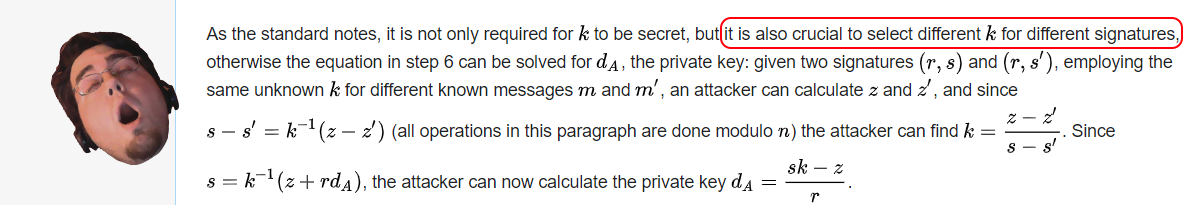Welcome to the Debugmen blog. We use this site to post tools, security findings, CTF writeups and anything else we find worthy of release to the public.

# ECDSA Implementation Review

## Overview

As the challenge name implies, this problem has to do with the given implementation of Elliptic Curve Digital Signature Algorithm (ECDSA).

They give us a python script that generates a private & public key, two randomly generated hashes to act as hashed data to digitally sign, the signatures of those hashes and finally an flag that’s been encrypted with AES using the private key generated for the ECDSA :

``````import ecdsa
import random
from Crypto.Cipher import AES
import binascii

def pad(m):
return m+chr(16-len(m)%16)*(16-len(m)%16)

gen = ecdsa.NIST256p.generator
order = gen.order()
secret = random.randrange(1,order)

pub_key = ecdsa.ecdsa.Public_key(gen, gen * secret)
priv_key = ecdsa.ecdsa.Private_key(pub_key, secret)

nonce1 = random.randrange(1, 2**127)
nonce2 = nonce1

# randomly generate hash value
hash1 = random.randrange(1, order)
hash2 = random.randrange(1, order)

sig1 = priv_key.sign(hash1, nonce1)
sig2 = priv_key.sign(hash2, nonce2)

s1 = sig1.s
s2 = sig2.s

print("r: " + str(sig1.r))
print("s1: " + str(s1))
print("s2: " + str(s2))
print("")
print("hashes:")
print(hash1)
print(hash2)
print("")
print("order: " + str(order))
print("")

aes_key = secret.to_bytes(64, byteorder='little')[0:16]

ptxt =  pad("flag{example}")
IV = b'\0\0\0\0\0\0\0\0\0\0\0\0\0\0\0\0'
cipher = AES.new(aes_key, AES.MODE_CBC, IV)
ctxt = cipher.encrypt(ptxt.encode('utf-8'))

print("Encrypted Flag:")
print(binascii.hexlify(ctxt))
``````

and the program output printing a bunch of the values:

``````r: 50394691958404671760038142322836584427075094292966481588111912351250929073849
s1: 26685296872928422980209331126861228951100823826633336689685109679472227918891
s2: 40762052781056121604891649645502377037837029273276315084687606790921202237960

hashes:
777971358777664237997807487843929900983351335441289679035928005996851307115
91840683637030200077344423945857298017410109326488651848157059631440788354195

order: 115792089210356248762697446949407573529996955224135760342422259061068512044369

Encrypted Flag:
b'f3ccfd5877ec7eb886d5f9372e97224c43f4412ca8eaeb567f9b20dd5e0aabd5'
``````

## Stealing the Private Key

Without much background in how ESDA works I looked up the wikipedia page for it and found that if you use the same k value, then the private key can be recovered.In this case, k is the `nonce1` and `nonce2` variables and they are both the same value. 🤔

Furthermore, the wikipedia page also tells you how to recover such private key in this situation. So all thats left to do is recover the private key and use that key as the key to AES decrypt the flag

``````import ecdsa
import random
from Crypto.Cipher import AES
import binascii
from ecdsa.numbertheory import inverse_mod
import IPython

# The vars given

r = 50394691958404671760038142322836584427075094292966481588111912351250929073849
s1 = 26685296872928422980209331126861228951100823826633336689685109679472227918891
s2 = 40762052781056121604891649645502377037837029273276315084687606790921202237960

h1 = 777971358777664237997807487843929900983351335441289679035928005996851307115
h2 = 91840683637030200077344423945857298017410109326488651848157059631440788354195

n = order = 115792089210356248762697446949407573529996955224135760342422259061068512044369

enc_flag = b'f3ccfd5877ec7eb886d5f9372e97224c43f4412ca8eaeb567f9b20dd5e0aabd5'

# Solve for k
z1=h1
z2=h2

# multiply with the inverse_mod to do 'division' in (mod `order`) space
k = (((z1 - z2) % order) * inverse_mod(s1-s2 ,order)) % order
print('k = ', k)

# mapping it to the vars from the original problem
nonce1 = nonuce2 = k

# Private Key i.e secret

secret = priv_key = (((s1*k) - z1) * inverse_mod(r, n)) % n
print('secret = ', secret)
aes_key = secret.to_bytes(64, byteorder='little')[0:16]

IV = b'\0\0\0\0\0\0\0\0\0\0\0\0\0\0\0\0'
cipher = AES.new(aes_key, AES.MODE_CBC, IV)
ctxt = cipher.decrypt(binascii.unhexlify(enc_flag))

print(ctxt)
print(binascii.hexlify(ctxt))
``````

and thus, the flag:

`flag{cRypt0_c4r3fully}`

## P.S.

To verify I properly recovered the private key, I inserted the values I calculated back into the original script and got the same signatures which means my key must be the correct.